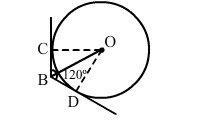# Two tangents BC and BD are drawn to a circle with centre O,`
Question:

Two tangents BC and BD are drawn to a circle with centre O, such that ∠CBD = 120°. Prove that OB = 2BC.Solution:

Here, $O B$ is the bisector of $\angle C B D$.

( $\mathrm{T}$ wo tangents are equally inclined to the line segment joining the centre to that point)

$\therefore \angle C B O=\angle D B O=\frac{1}{2} \angle C B D=60^{\circ}$

$\therefore$ From $\Delta B O D, \angle B O D=30^{\circ}$

Now, from right - angled $\Delta B O D$,

$\frac{B D}{O B}=\sin 30^{\circ}$

$\Rightarrow \frac{B D}{O B}=\frac{1}{2}$

$\Rightarrow O B=2 B D$

$\Rightarrow O B=2 B C$ (Since tangents from an external point are equal, i. e. $B C=B D$ )

$\therefore O B=2 B C$